﻿ 基于(17, 9)平方剩余码的广义LDPC码构造及性能研究文章快速检索 高级检索
 重庆邮电大学学报(自然科学版)2018, Vol. 30Issue (6): 797-802  DOI: 10.3979/j.issn.1673-825X.2018.06.0100

### 引用本文LI Xiya, LI Yong. Research on design and performance of GLDPC codes base on (17, 9) quadratic residue codes[J]. Journal of Chongqing University of Posts and Telecommunications (Natural Science Edition), 2018, 30(6): 797-802. DOI: 10.3979/j.issn.1673-825X.2018.06.010.### 文章历史

Research on design and performance of GLDPC codes base on (17, 9) quadratic residue codes
LI Xiya, LI YongChongqing Key Laboratory of Mobile Communications Technology, Chongqing University of Posts and Telecommunications, Chongqing 400065, P. R. China
Foundation Items: The National Natural Science Foundation of China (61771081)
Abstract: The check node of low density parity check(LDPC)uses single parity check(SPC) code, while generalized LDPC(GLDPC)codes with better performance can be constructed by replacing the SPC code in LDPC codes with a component codes that has stronger error correction capability. In view of this, this paper uses (17, 9) quadratic residue(QR) code as the component codes to replace the SPC code to construct a quadratic residue-GLDPC(QR -GLDPC) code. Firstly, this paper studies the construction of GLDPC codes and QR code, and studies the decoding algorithm of GLDPC code. Based on those, a construction method of GLDPC codes based on (17, 9) QR code is proposed and then its performance is also studied. Finally, we simulate the GLDPC code and the traditional LDPC code, different code length of GLDPC at the same code rate, the same code length of GLDPC at different code rate. The simulation results indicate that compared with LDPC codes at the same bit rate, GLDPC codes based on (17, 9) QR codes achieve better performance in terms of the bit error rate and the decoding convergence speed.
Keywords: quadratic residue code    generalized LDPC(GLDPC)    construct    replaced    convergence
0 引言

1960年代初，Gallager首次提出了低密度奇偶校验(low-density parity check, LDPC)码的概念，它是一类优秀的纠错码，具有较好的译码性能。近年来，LDPC码的研究获得了较大关注，在迭代译码情况下，LDPC码性能可以逼近香农极限。传统LDPC码尽管有着较高的编码增益，但其错误地板和译码复杂度往往较高。为了获得更低的错误地板，1999年，Lentmaier和Zigangirov首次构造了以汉明码作为分量码替换LDPC码中单奇偶校验(single parity-check, SPC)码的广义LDPC(generalized LDPC, GLDPC)码，其仿真性能相比同码率下的LDPC码表现更加优秀。同时也在理论上证明了GLDPC码能够以较快的收敛速度取得较低的错误地板

GLDPC码是对LDPC码概念的进一步拓展。通过观察GLDPC码的Tanner图发现，GLDPC码的变量节点和校验节点使用更为灵活，不再局限于传统的LDPC码的变量节点和SPC节点，这也使得GLDPC码的译码方式更为灵活。目前常见的替换SPC码的分量码有BCH(Bose，Ray-Chaudhuri，Hocquenghem)，里德-所罗门(Reed-Solomon, RS)等码，它们的纠错能力比SPC码强，构造出的GLDPC码的性能也比同码率下的LDPC码更加优秀。然而这种为了增强校验节点约束而引入了更多的校验方程的方式，使得GLDPC码的码率大大降低。为了减少码率损失，在设计GLDPC码时就必须减少LDPC母码(也称全局码)中被替换的校验节点的数量，但这反过来又会影响码字的性能。鉴于此，必须采用比传统的汉明码、BCH码更强大的码型作分量码，从而使只替换母码中少部分校验节点即可设计出性能优异的GLDPC码。本文针对分量码为平方剩余(quadratic residue, QR)码的GLDPC码提出了一种构造方法，构造了一个QR-GLDPC码。

QR码是BCH码的一个子类，有着比传统的循环码更大的最小汉明距离。1957年，QR码由Prange首次提出，因具有完美的代数结构，受到众多代数编码学家的青睐，这极大地加快了QR码用于纠错应用的研究进展。

1 GLDPC码和QR码介绍 1.1 GLDPC码的定义

GLDPC码的校验矩阵构造由2部分组成，分别是校验母矩阵HGLDPC和分量码校验矩阵HCPT，其中，HGLDPCm0n0列。基于以上2种矩阵扩展而成的GLDPC码的校验矩阵为HGLDPC, 有MN列，其中N=n0。如果HGLDPC是满秩的，则GLDPC码的码率R可表示为

 $R = \frac{{N - M}}{N} = 1 - \frac{M}{N}$ (1)
1.2 GLDPC码的Tanner图表示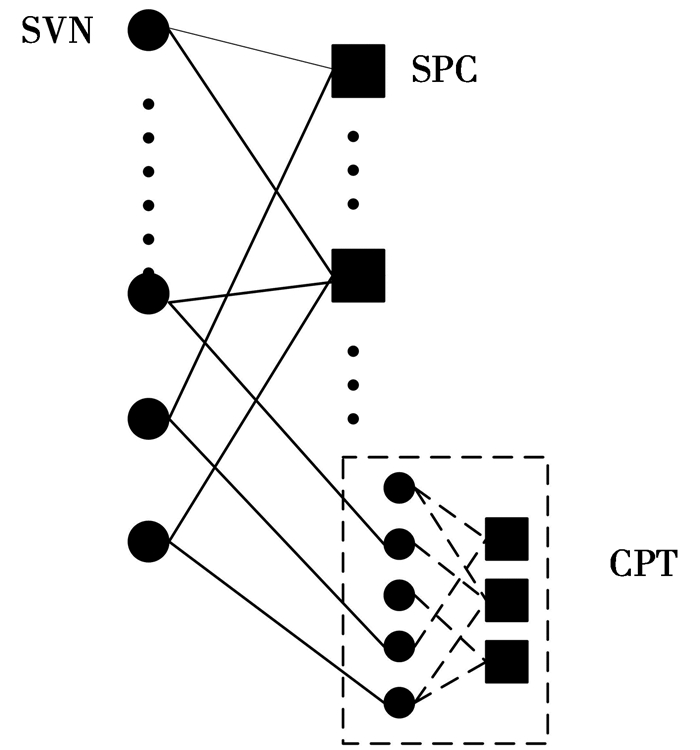图 1 GLDPC码的Tanner图 Figure 1 Tanner graph of GLDPC codes
1.3 QR码介绍

QR码是码率略大于1/2的BCH码的一个子类，在同等码长下，比RS码、汉明码等具有更优异的纠错性能。

 $g\left( x \right) = {g_0} + {g_1}X + \cdots + {g_{n - k}}{X^{n - k}}$ (2)

(2) 式中：n是码字长度，k=(n+1)/2是码字信息位的长度；d是最小汉明距离。假设h(x)是QR码的校验多项式，由文献可知Xn+1的一个因式是g(x)，即

 $g\left( x \right) \cdot h\left( x \right) = {X^n} + 1$ (3)

(3) 式中，h(x)可以表示为如下的多项式形式

 $h\left( X \right) = {h_0} + {h_1}X + \cdots + {h_k}{X^k}$ (4)

(4) 式中，h0=hk=1。经计算，可以得到(n-k)个方程式。

 $\sum\limits_{i = 1}^k {{h_i}{v_{n - i - j}}} = 0,\;\;\;\;1 \le j \le n - k$ (5)

(5) 式中，vn-i-j表示码字中的一位。因此，可以推导出h(x)的反多项式，即

 ${X^k}h\left( {{X^{ - 1}}} \right) \buildrel \Delta \over = {h_k} + {h_{k - 1}}X + {h_{k - 2}}{X^2} + \cdots + {h_0}{X^k}$ (6)

 ${\mathit{\boldsymbol{H}}_{{\rm{QR}}}} = \left[ {\begin{array}{*{20}{c}} {{h_k}}&{{h_{k - 1}}}& \cdots &{{h_0}}&0& \cdots &0&{}\\ 0&{{h_k}}&{{h_{k - 1}}}& \cdots &{{h_0}}&0& \cdots &0\\ \vdots&\vdots&\vdots &{}& \vdots &{}&{}&{}\\ 0&0& \cdots &0&{{h_k}}&{{h_{k - 1}}}& \cdots &{{h_0}} \end{array}} \right]$ (7)

 $g\left( x \right) = {x^8} + {x^5} + {x^4} + {x^3} + 1$ (8)

 ${x^9}h\left( {{x^{ - 1}}} \right) = {x^9} + {x^6} + {x^5} + {x^4} + {x^3} + 1$ (9)

 ${\mathit{\boldsymbol{H}}_{{\rm{QR}}}} = \left[ {\begin{array}{*{20}{c}} 1&0&0&1&1&1&1&0&0&1&0&0&0&0&0&0&0\\ 0&1&0&0&1&1&1&1&0&0&1&0&0&0&0&0&0\\ 0&0&1&0&0&1&1&1&1&0&0&1&0&0&0&0&0\\ 0&0&0&1&0&0&1&1&1&1&0&0&1&0&0&0&0 \end{array}} \right]$

2 GLDPC码构造和译码 2.1 GLDPC码的构造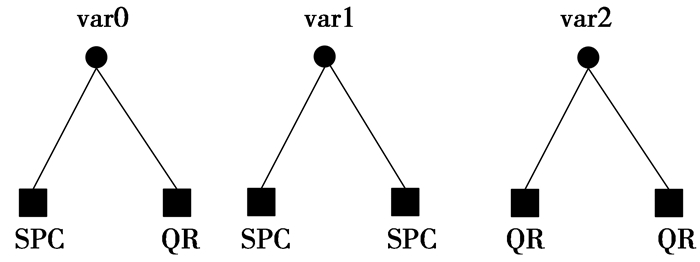图 2 3种类型的变量节点 Figure 2 Three types of variable nodes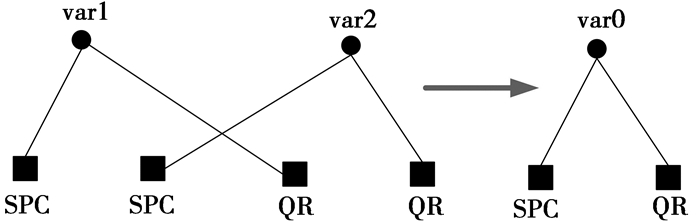图 3 交换边的原理 Figure 3 Principle of exchange sides

2.2 GLDPC码的译码

 $LL{R_{{\rm{ch}}}}\left( i \right) = \frac{{4\sqrt {{E_{\rm{s}}}} }}{{{N_0}}} \cdot {r_i},i = 0,1,2, \cdots ,N - 1$ (10)

 $LLR_{{t_{{\rm{exter}}}}}^l\left( i \right) = {y_{{\rm{output}}}} - {y_{{\rm{input}}}}$ (11)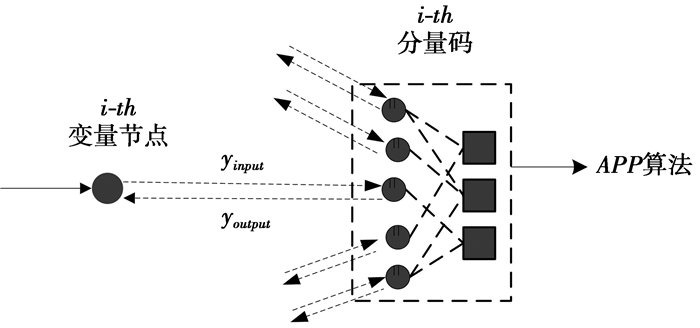图 4 变量节点i从第t个分量码获得的外信息 Figure 4 External information obtained of the variable node ifrom the tcomponent code

(11) 式中，yinput是变量节i点传递给分量码t的置信值，即(12)式中的置信值LLRtl(i)，yinput=LLRtl(i)，当l=1时，yinput等于变量节点i的初始值LLRch(i)；youtput是分量码t传递给变量节点i的值，当分量码收集到所有相邻的变量节点信息后，通过APP译码算法处理得到一组输出值，然后传递给所有相邻的变量节点，youtput即为传递给变量节点i的其中一个输出值。

 $LLR_t^l\left( i \right) = LL{R_{{\rm{ch}}}}\left( i \right) + \sum\limits_{t' \in T\left( i \right)\backslash t} {LLR_{{{t'}_{{\rm{exter}}}}}^l\left( i \right)}$ (12)

 $LL{R^L}\left( i \right) = LL{R_{{\rm{ch}}}}\left( i \right) + \sum\limits_{t \in T\left( i \right)} {LLR_{{t_{{\rm{exter}}}}}^L\left( i \right)}$ (13)

 ${{\hat v}_i} = \left\{ \begin{array}{l} 0,\;\;若\;LL{R^L}\left( i \right) > 0\\ 1,\;\;其他 \end{array} \right.\;\;\;i = 0,1, \cdots ,N - 1$ (14)

2.3 分量码的APP译码算法

APP译码算法是一种适用于线性分组码的最大后验概率译码算法。下面介绍该算法以及算法中相关符号的含义。

 ${\mathit{\boldsymbol{H}}^{\rm{T}}} = \left( {\begin{array}{*{20}{c}} {{\mathit{\boldsymbol{h}}_1}}\\ {{\mathit{\boldsymbol{h}}_2}}\\ \vdots \\ {{\mathit{\boldsymbol{h}}_N}} \end{array}} \right)$ (15)

(15) 式中，hn=(hn1, hn2, …, hn(N-K))，1≤nN

 $\mathit{\boldsymbol{H}}_{\left[ {1,n} \right]}^{\rm{T}} = \left( {\begin{array}{*{20}{c}} {{\mathit{\boldsymbol{h}}_1}}\\ {{\mathit{\boldsymbol{h}}_2}}\\ \vdots \\ {{\mathit{\boldsymbol{h}}_n}} \end{array}} \right)$ (16)

P(rn|v)表示先验概率，P(vn|r)表示后验概率。在上述GLDPC码译码算法中，可根据分量码中各变量节点的输入值yinput=LLRtl(i)=ln(P(rn|vn=0)/P(rn|vn=1))计算得到P(rn|vn=0)=(1/(1+ eyinput))，因此，P(rn|vn=1)=1-P(rn|vn=0)。相应的输出值youtput=ln(P(vn=0|r)/P(vn=1|r))，1≤nN。APP译码算法的具体步骤如下。

 $\begin{array}{*{20}{c}} {\mu \left( {{\bf{s}},n} \right) = \mu \left( {{\bf{s}},n - 1} \right)P\left( {{r_n}\left| {{v_n} = 0} \right.} \right) + }\\ {\mu \left( {{\bf{s}} + {\mathit{\boldsymbol{h}}_n},n - 1} \right)P\left( {{r_n}\left| {{v_n} = 1} \right.} \right)} \end{array}$

 $P\left( {{v_n} = 0\left| {\mathit{\boldsymbol{r}},\mathit{\boldsymbol{v}}} \right.} \right) = \frac{{\frac{{P\left( {{r_n}\left| {{v_n} = 0} \right.} \right)}}{{P\left( {{r_n}\left| {{v_n} = 1} \right.} \right)}} - \frac{{\mu \left( {{\mathit{\boldsymbol{h}}_n},N} \right)}}{{\mu \left( {{\bf{0}},N} \right)}}}}{{\frac{{P\left( {{r_n}\left| {{v_n} = 0} \right.} \right)}}{{P\left( {{r_n}\left| {{v_n} = 1} \right.} \right)}} - \frac{{P\left( {{r_n}\left| {{v_n} = 1} \right.} \right)}}{{P\left( {{r_n}\left| {{v_n} = 0} \right.} \right)}}}}$

3 仿真结果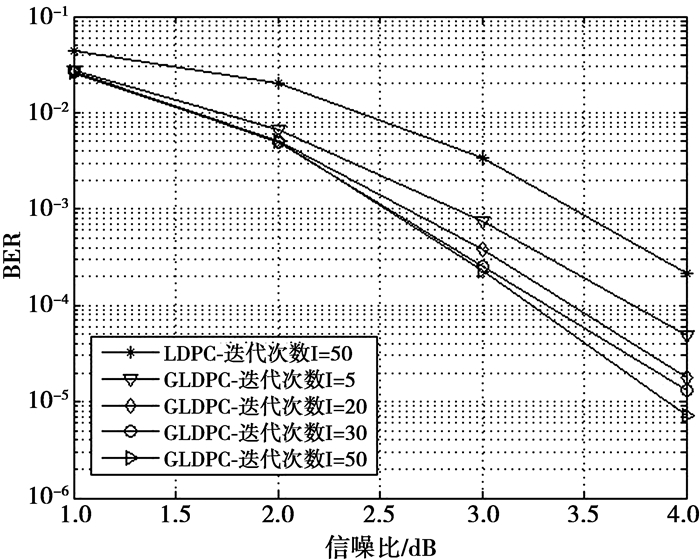图 5 GLDPC码与LDPC码性能 Figure 5 Performance of GLDPC and LDPC图 6 同码率不同码长、同码长不同码率GLDPC码性能比较 Figure 6 Comparison of the performance of different length of GLDPC at the same rate and the same length of GLDPC at the different rate

4 结论

  GALLAGER R G. Low-density parity-check codes[J]. Journal of Circuits & Systems, 2008, 8(1): 3-26.  LENTMAIER M, ZIGANGIROV K S. On generalized low-density parity-check codes based on Hamming component codes[J]. IEEE Communications Letters, 1999, 3(8): 248-250. DOI:10.1109/4234.781010  DJORDJEVIX IB, MILENKOVIC O, VASIC B. Generalized Low Density Parity Check Codes for optical communication systems[J]. Journal of Lightwave Technology, 2015, 23(5): 1939-1946.  SOLOPERTO R, GARRAMMONE G, LIVA G, et al. Quasi-Cyclic Doubly Generalized LDPC Codes[C]// Vehicular Technology Conference. New York: IEEE Press, 2013: 1-5. http://ieeexplore.ieee.org/xpls/abs_all.jsp?arnumber=6692727  MILADINOVIC N, FOSSORIER M. Generalized LDPC codes with Reed-Solomon and BCH codes as component codes for binary channels[C]//IEEE Global Telecommunications Conference. New York: IEEE Press, 2005: 6. http://ieeexplore.ieee.org/xpls/icp.jsp?arnumber=1577850  CHENG Gaoming, LI Yong, DONG Can, ZHANG Xinqiu, et al. A fast algebraic decoding algorithm of the(71, 36, 11) quadratic residue code[J]. Journal of Chongqing University of Posts & Telecommunications, 2015, 27(6): 781-785.  林舒, 科斯特洛. 差错控制编码[M]. 北京: 机械工业出版社, 2007. LIN Shu, JR D J C. Error Control Coding[M]. Beijing: China Machine Press, 2007.  HE Y, YANG J, SUN G. Doubly-generalized LDPC codes with SPC codes as super variable nodes[C]// Wireless Communications and NETWORKING Conference. New York: IEEE Press, 2015: 1072-1077. http://ieeexplore.ieee.org/xpls/abs_all.jsp?arnumber=7127618  JOHANSSON T, A K. A simple one sweep algorithm for optimal APP symbol decoding of linear block codes[C]// IEEE International Symposium on Information Theory, 1998. Proceedings. New York: IEEE Press, 2002: 231. http://ieeexplore.ieee.org/iel4/5721/15310/00708835.pdf  DIOUF M, DECLERCQ D, OUYA S, et al. A PEG-like LDPC code design avoiding short trapping sets[C]// IEEE International Symposium on Information Theory. I New York: IEEE Press, 2015: 1079-1083.